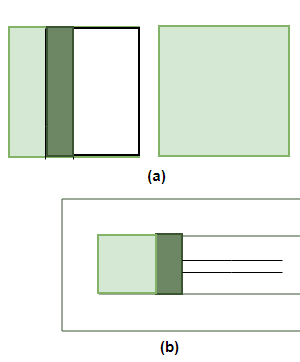Thermodynamic State Variables and Equation of State

• Last Updated : 20 Oct, 2021

The branch of thermodynamics deals with the process of heat exchange by the gas or the temperature of the system of the gas. This branch also deals with the flow of heat from one part of the system to another part of the system. For systems that are present in the real world, there are some parameters that can define their state. These are called thermodynamic variables. These variables and the equations related to them are essential for us to study and predict the behavior of such systems.

Thermodynamic State Variables and State Equation

A system is said to be in a thermodynamics state of equilibrium if the macroscopic variables that change the state of the system do not change over time. These macroscopic variables include pressure, temperature, mass, and composition that does not change with time. For example, gas is stored inside a container that is completely insulated from its surroundings, with fixed values of pressure, volume, temperature, mass, and composition that do not change with time, is in a state of equilibrium.

Every equilibrium state of a thermodynamic system can be described by specific values of macroscopic variables. These variables are also called state variables.

The equilibrium of a gas can be described by its pressure, temperature, volume, and mass. It is not necessary that a thermodynamic system is always in equilibrium. For example, if gas at equilibrium is allowed to expand, it does not remain in thermodynamic equilibrium. The figure below shows the expansion of gases when they are left to expand:In short, thermodynamics variables describe the state of the system at equilibrium. These various state variables are not necessarily independent.

These variables can be divided into two types:

1. Extensive Variables
2. Intensive Variables

Extensive Variables: These variables are the state variables that indicate the size of the system. For example, Volume can be considered an extensive variable because it gives us an idea about the size of the system.

Intensive Variables: These variables are the state variables that do not give us any information about the size of the system but indicate different information about the system. Examples of such variables are pressure, temperature, etc.

State Equation

State Equation describes the relationship between state variables of a thermodynamic system. The equation of state is completely defined in terms of pressure, temperature and volume. For example, in the case of ideal gases. The state equation becomes,

PV = RT

or

PV = Constant

In the case of an isothermal process,

P1V1 = P2V2

These equations of state are for ideal gases.

Sample Problems

Question 1: In an isothermal thermodynamic process, initial pressure and volume are 106 N/m2 and 3m3 respectively. Now, the pressure of the container is doubled. Find the volume.

In the case of an isothermal process,

P1V1 = P2V2

Given:

P1 = 106, P2 = 2 × 106 and V1 = 3

Plugging the values in the equation,

P1V1 = P2V2

⇒ 106 × 3 = 2 × 106 × V2

⇒ 3 = 2 × V2

⇒ 1.5 m3 = V2

Question 2: In an isothermal thermodynamic process, initial pressure and volume are 5 × 106 N/m2 and 6m3 respectively. Now, the pressure of the container is halved. Find the volume.

In the case of an isothermal process,

P1V1 = P2V2

Given:

P1 = 5 × 106, P2 = 2.5 × 106 and V1 = 3

Plugging the values in the equation,

P1V1 = P2V2

⇒ 5 × 106 × 3 = 2.5 × 106 × V2

⇒ 15 = 2.5 × V2

⇒ 6m3 = V2

Question 3: In a thermodynamic process, the volume remains constant. Initial pressure and temperature are 5 × 106 N/m2 and 100K respectively. Now, the pressure of the container is halved. Find the new temperature.

In the case of an isothermal process,

P1T2 = P2T1

Given:

P1 = 5 × 106, P2 = 2.5 × 106 and T1 = 100 K

Plugging the values in the equation,

P1T2 = P2T1

⇒ 5 × 106 × T2 = 2.5 × 106 × 100

⇒ T2 = 0.5 × 100

⇒ 50 K = T2

Question 4: In a thermodynamic process, the volume remains constant. Initial pressure and temperature are 106 N/m2 and 250K respectively. Now, the pressure of the container is increased four times. Find the new temperature.

In the case of an isothermal process,

P1T2 = P2T1

Given:

P1 = 106, P2 = 4 × 106 and T1 = 250 K

Plugging the values in the equation,

P1T2 = P2T1

⇒ 1 × 106 × T2 = 4 × 106 × 100

⇒ T2 = 4 × 100

⇒ 400K = T2

Question 5: In a thermodynamic process, the pressure remains constant. Initial volume and temperature are 5m3 and 250K respectively. Now, the volume of the container is increased two times. Find the new temperature.

In the case of an isothermal process,

V1T2 = V2T1

Given:

V1 = 5 m3, V2 = 10  m3 and T1 = 250 K

Plugging the values in the equation,

V1T2 = V2T1

⇒ 5 × T2 = 10 × 250

⇒ T2 = 500

⇒ 500K = T2

My Personal Notes arrow_drop_up